﻿ 施工升降机单肢稳定性计算校核 Calculation and Verification of Single Limb Stability of Construction Lifts

Mechanical Engineering and Technology
Vol.06 No.04(2017), Article ID:22492,9 pages
10.12677/MET.2017.64037

Calculation and Verification of Single Limb Stability of Construction Lifts

Jianwei Liu1, Jiyong Wang1, Lei Cai2, Qi Zuo1

1School of Mechanical and Electrical Engineering, Shandong Jianzhu University, Jinan Shandong

2Jinan City Engineering Quality and Safety Production Supervision Station, Jinan ShandongReceived: Oct. 9th, 2017; accepted: Oct. 23rd, 2017; published: Oct. 30th, 2017ABSTRACT

In the design of the construction elevator, the problem of insufficient attention to the calculation of the stability of the single limbs is found, which leads to the fact that only the stability stress of the rail frame is less than the allowable stress in the design. In the course of use, stable phenomenon, according to GB26557-2011 “hanging cage with vertical guide the dual-use construction of the lift,” the relevant provisions of the comparison through the rails under the conditions of the load should be calculated under different load combinations, put forward the exception of the safety device. The most dangerous working conditions of the single limb are analyzed and compared with all the dangerous sections of the rail frame under the most dangerous conditions. It is determined that the cage is located in the middle of the guide rail frame and the bottom frame and the bottom. The cross section of the middle section of the middle frame is the most dangerous section, and the verification method of the single limb stability calculation under the most dangerous cross section of the most dangerous condition is given. Taking the SC200/200 construction lifts as an example, the stability of the single limbs is checked, which explains the reason why the construction lifts are widely used and the instability of the rails after the impact is large.

Keywords:Construction Lift, Guide Rail, GB26557-2011, Hanging Cage

1山东建筑大学机电工程学院，山东 济南

2济南市工程质量与安全生产监督站，山东 济南1. 引言

2. 施工升降机结构

3. 导轨架受力工况分析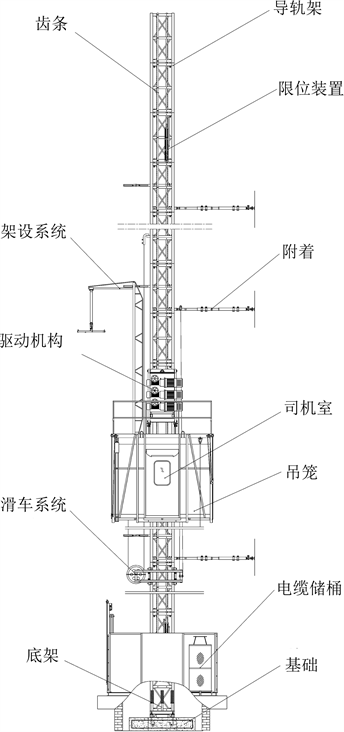Figure 1. Construction lifts structural drawings

3.1. Ia正常使用工况与IIIa异常情况的力工况

3.2. Ia正常使用工况与Ⅸ安装工况

3.3. Ia正常使用工况与Ⅳa异常情况下安全装置作用工况

3.4. IIa吊笼正常装载工况

3.5. VI偶尔不使用工况

4. 导轨架最危险截面分析

4.1. 导轨架受力与力矩

1) N为导轨架危险截面处的压力：

$N={G}_{额载}+{G}_{机构}+{G}_{司机}+{G}_{吊笼}+{G}_{导轨架}+{G}_{附墙架}$ (1)

2) MHx为风载荷在x方向(垂直附墙架的方向)分别作用在导轨架、吊笼、驱动机构上产生的弯矩和：

${M}_{Hx}={M}_{风\ast 导轨架}+{M}_{风\ast 吊笼}+{M}_{风\ast 机构}$ (2)

3) ${M}_{垂直度}$ 为导轨架垂直度产生的弯矩，N∙m，计算时要求导轨架垂直度误差至少为0.5˚。

4) ${M}_{附墙架\ast y}$ 为所求危险截面以上附墙架作用在导轨架上的力在y方向(垂直附墙架的方向)上对导轨架作用，产生弯矩，N∙m。

5) Mox${G}_{额载}$${G}_{机构}$${G}_{司机}$${G}_{吊笼}$ 分别对导轨架在x方向作用产生的弯矩和，N∙m。

6) Moy${G}_{额载}$${G}_{机构}$${G}_{司机}$${G}_{吊笼}$ 分别对导轨架在y方向作用产生的弯矩和，N∙m。

4.2. 危险截面

4.3. 危险截面1与危险截面2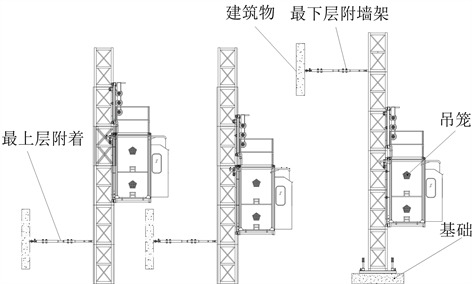Figure 2. Construction lift hazard section

4.4. 危险截面1与危险截面3

5. 单肢稳定性计算校核

5.1. 吊笼滚轮产生的压力

${N}_{滚轮}=\left({M}_{ox}-\left({G}_{额载}+{G}_{机构}+{G}_{司机}+{G}_{吊笼}\right)×\mu ×{l}_{2}\right)/{l}_{1}/4+{F}_{风\ast 吊笼}/4$ (3)

5.2. 结构计算简图

5.3. 吊笼滚轮产生的弯矩

${\delta }_{11}{X}_{1}+{\Delta }_{1p}=0$ (4)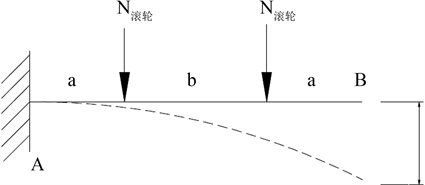Figure 3. Simplified model structure calculation diagram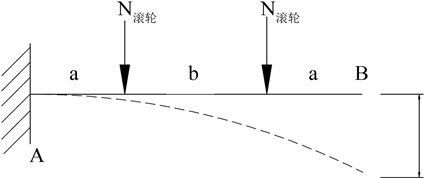Figure 4. Roller force displacement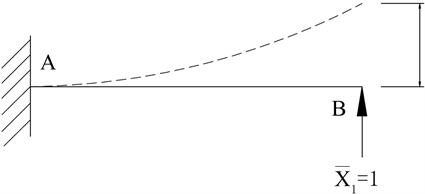Figure 5. Unit force displacement

${\Delta }_{1p}={\int }_{0}^{l}\frac{\stackrel{¯}{M}{M}_{p}}{EI}\text{d}x$ (5)

${\delta }_{11}={\int }_{0}^{l}\frac{\stackrel{¯}{{M}_{1}}{M}_{p1}}{EI}\text{d}x$ (6)

${M}_{滚轮}=X×a$ (7)

5.4. 施工升降机导轨架主肢应力

$F=\frac{N}{4}+\frac{{M}_{ox}+{M}_{垂直度}+{M}_{Hx}}{2×0.65}+\frac{{M}_{oy}+{M}_{附墙架\ast y}}{2×0.65}$ (8)

$\sigma =\frac{F}{\phi A}+\frac{{M}_{滚轮}}{{W}_{主肢}}<\left[\sigma \right]$ (9)

6. 计算实例

6.1. 施工升降机参数

SC200/200施工升降机导轨架最大高度150 m，最大悬臂高度7.5 m，单笼额定起重量20 KN，吊笼及附属结构自重12.9 KN，驱动机构自重6 KN，司机重0.8 KN。计算截面为IVα异常情况下安全装置作用下，两额定起重量吊笼分别在上端和底架与最下附墙架中间时，底架与最下附墙架中间的导轨架处截面。选择防坠器时，要选择质量高的机构以确保载荷冲击系数在1.58以内。

6.2. 导轨架单肢稳定性校核计算

$N=221280\text{\hspace{0.17em}}\text{N}$ (10)

${M}_{ox}=68724\text{\hspace{0.17em}}\text{N}\cdot \text{m},\text{\hspace{0.17em}}\text{\hspace{0.17em}}{M}_{oy}=4992\text{\hspace{0.17em}}\text{N}\cdot \text{m}$ (11)

${M}_{垂直度}=12552\text{\hspace{0.17em}}\text{N}\cdot \text{m}$ (12)

${M}_{附墙架\ast y}=4214\text{\hspace{0.17em}}\text{N}\cdot \text{m}$ (13)

${M}_{Hx}=4940\text{\hspace{0.17em}}\text{N}\cdot \text{m}$ (14)

${l}_{0}=0.7×700=490\text{\hspace{0.17em}}\text{mm}$ (15)

$\lambda =\frac{{l}_{0}}{{r}_{\mathrm{min}}}=\frac{490}{25.3}=19.36$ (16)

${N}_{滚轮}=5471\text{\hspace{0.17em}}\text{N}$ (17)

${M}_{滚轮}=982\text{\hspace{0.17em}}\text{N}\cdot \text{m}$ (18)

$F=128724\text{\hspace{0.17em}}\text{N}$ (19)

$\sigma =187.2\text{\hspace{0.17em}}\text{MPa}<\left[\sigma \right]=188\text{\hspace{0.17em}}\text{MPa}$ (20)

7. 结论

1) 超速安全装置作用下载荷冲击系数在1.58以内时，SC200/200施工升降机导轨架单肢稳定性的应力小于许用应力，满足稳定性的要求。

2) 导轨架在受到较大的冲击后时，导轨架将发生单肢失稳，从而导致导轨架整体失稳。目前导轨架采用的材料从单肢稳定的角度已无冗余，不可根据整体稳定的计算结论减小材料。

3) 在施工升降机导轨架的设计计算时，不仅仅要计算强度、结构整体稳定性方面的要求，单肢稳定性的问题更要予以重视。

Calculation and Verification of Single Limb Stability of Construction Lifts[J]. 机械工程与技术, 2017, 06(04): 302-310. http://dx.doi.org/10.12677/MET.2017.64037

1. 1. 胡燕东. 工程起重机中超静定桁架结构的弹性稳定性分析[D]: [硕士学位论文]. 哈尔滨: 哈尔滨工业大学, 2010.

2. 2. 肖洪涛, 张磊, 陈浩, 王红格, 郑可勇. 齿轮齿条式永久升降机浅析及应用[J]. 建筑机械化, 2017, 38(7): 34-36.

3. 3. GB/T 26557-2011, 吊笼有垂直导向的人货两用施工升降机[S].

4. 4. 王积永, 张青, 沈孝琴, 等. 起重机械钢结构设计[M]. 北京: 化学工业出版社, 2011.

5. 5. 李家宝, 洪范文, 童桦, 等. 结构力学[M]. 北京: 高等教育出版社, 2005.